• 在统计实践中，人们经常需要对样本资料进行各种各样的分类，以便分析研究。如果对样本资料按照两个指标变量进行...这种对列联表中两分类变量是否独立的检验，也是假设检验的一个重要内容，称为列联表分析列联表检验。
• 高维列联表分析流程 目录 第一章 绪论 7 1.1 问题研究背景 7 1.2 数据来源 7 1.3 研究意义 1 第二章 高维列联表独立性检验 2 2.1 高维列联表的相互独立性检验 2 2.2 高维列联表的边缘独立性检验 2 2.3 高维列联表的...
第一篇
关于定性数据分析
上学期选修了定性数据分析这门课程，教材是王静龙、梁小筠的定性数据分析。
高维列联表分析流程
目录
第一章 绪论	7
1.1 问题研究背景	7
1.2 数据来源	7
1.3 研究意义	1
第二章 高维列联表独立性检验	2
2.1 高维列联表的相互独立性检验	2
2.2 高维列联表的边缘独立性检验	2
2.3 高维列联表的条件独立性检验	3
2.3.1层属性“地区”给定时的条件独立性检验	3
2.3.2行属性“性别”给定时的条件独立性检验	4
第三章 高维列联表相合性检验	5
3.1 高维列联表的压缩相合性检验	5
3.2 高维列联表分层相合性检验	5
3.3 高维列联表条件相合性检验（Cochran-Mantel-Haenszel检验）	7
3.2 高维列联表相合齐次性检验（Breslow-Day检验）	8
第四章 结论与建议	10
4.1 结论	10
4.2 相关建议	11
参考文献	12
直接上代码给需要的人（R）
// An highlighted block
附录4
###################定性数据统计分析论文##########
#####三维列联表的压缩与分层
##三维列联表压缩函数，输入为三维列联表(行*列*层)数组，按层压缩，得到边缘表
compress=function(x){
x_compress=matrix(0,nrow = dim(x),ncol = dim(x))#构建一个与边缘表相同大小的0矩阵
for (k in 1:dim(x)) {##dim(z)#数组z的层数
x_compress=x_compress+x[,,k]#x[,,k]第k层数据矩阵
}##dim(z)#数组z的层数
return(x_compress)#返回边缘表
}

##三维列联表分层与相合性检验函数，输入为三维列联表(行*列*层)数组，按层分层，得到部分表和检验结果
layering=function(x){
for (k in 1:dim(x)) {##dim(z)#数组z的层数
x_layer=x[,,k]#x[,,k]第k层数据矩阵
print(x_layer)#打印部分表
congruence_test(x_layer,alternative="greater")###相合性检验，H1:正相合
cat('\n')
}##dim(z)#数组z的层数
}
congruence_test=function(x,alternative="")
#适用于列联表的相合性度量与检验问题
#x为列联表矩阵,alternative对应于备择假设
{
n=sum(x)
G=0;H=0
r=nrow(x)
c=ncol(x)
r1=r-1;c1=c-1
#计算G:
for (i in 1:r1){
for (j in 1:c1){
G=G+x[i,j]*sum(x[(i+1):r,(j+1):c])
}
}
#计算H：
for (i in 1:r1){
for (j in 2:c){
H=H+x[i,j]*sum(x[(i+1):r,1:(j-1)])
}
}
#计算z,TA,TB,TAB:
z=G-H
TA=sum(rowSums(x)*(rowSums(x)-1)/2)
TB=sum(colSums(x)*(colSums(x)-1)/2)
#TAB=G+H+TA+TB-n*(n-1)/2
Cn2=n*(n-1)/2
######相合性度量######
#计算各系数的值
Kendall_tau=z/sqrt((Cn2-TA)*(Cn2-TB))###Kendall-tau系数
Gamma=(G-H)/(G+H)######Gamma系数
#####Somers-d系数：
d_BA=(G-H)/(Cn2-TA)###列联表为2*c,列属性B依赖于行属性A，用d_B|A度量相合性
d_AB=(G-H)/(Cn2-TB)###列联表为r*2,行属性A依赖于列属性B，用d_A|B度量相合性

#######相合性检验######
#sigma平方的近似计算公式,表示sigma的平方
sigma_2=(n^3-sum(rowSums(x)^3))*(n^3-sum(colSums(x)^3))/(9*n^3)
#构建检验统计量：U统计量
U=z/sqrt(sigma_2)
if(alternative=="two.side")
{p_value=1-pchisq(U^2, 1)}
else
{
if(alternative=="greater")####greater：备择假设为正相合
{p_value=pnorm(-U)}
else if(alternative=="less")####less:备择假设为负相合
{p_value=pnorm(U)}
}
cat('关于相合性度量的相关系数：\n')
cat('G=',G,'\n')
cat('H=',H,'\n')
cat('z=',z,'\n')
cat('T_A=',TA,'\n')
cat('T_B=',TB,'\n')
cat('Kendall_tau=',Kendall_tau,'\n')
cat('Gamma=',Gamma,'\n')
cat('d_B|A=',d_BA,'\n')
cat('d_A|B=',d_AB,'\n\n')
cat('相合性检验:\n')
cat('检验统计量的值U=',U,'\n')
cat('p_value=',p_value,'\n')
}
##高维(三维)列联表的条件独立性检验
Conditional_independence_test=function(x){
Likelihood_ratio_layer=0
Likelihood_ratio_row=0
Likelihood_ratio_col=0
#层属性C给定时计算似然比检验统计量的值
#原假设H0：层属性C给定后行属性A与列属性B条件独立，备择假设H1：层属性C给定后行属性A与列属性B不条件独立
for (k in 1:dim(x)) {##dim(z)#数组z的层数
for (i in 1:dim(x)) {##dim(z)#数组z的层数
for (j in 1:dim(x)) {
Likelihood_ratio_layer=Likelihood_ratio_layer+(-2*x[i,j,k]*log(sum(x[i,,k])*sum(x[,j,k])/(sum(x[,,k])*x[i,j,k])))
}
}
}
#层属性C给定时计算条件独立性检验的p值
p_value_layer=1-pchisq(Likelihood_ratio_layer, dim(x)*(dim(x)-1)*(dim(x)-1))

#行属性A给定时计算似然比检验统计量的值
#原假设H0：行属性A给定后层属性C与列属性B条件独立，备择假设H1：行属性A给定后层属性C与列属性B不条件独立
for (i in 1:dim(x)) {
for (j in 1:dim(x)) {
for (k in 1:dim(x)) {
Likelihood_ratio_row=Likelihood_ratio_row+(-2*x[i,j,k]*log(sum(x[i,,k])*sum(x[i,j,])/(sum(x[i,,])*x[i,j,k])))
}
}
}
#行属性A给定时计算条件独立性检验的p值
p_value_row=1-pchisq(Likelihood_ratio_row, dim(x)*(dim(x)-1)*(dim(x)-1))

#列属性B给定时计算似然比检验统计量的值
#原假设H0:列属性B给定后行属性A与层属性C条件独立，备择假设H1：列属性B给定后行属性A与层属性C不条件独立
for (j in 1:dim(x)) {
for (i in 1:dim(x)) {
for (k in 1:dim(x)) {
Likelihood_ratio_col=Likelihood_ratio_col+(-2*x[i,j,k]*log(sum(x[i,j,])*sum(x[,j,k])/(sum(x[,j,])*x[i,j,k])))
}
}
}
#列属性B给定时计算条件独立性检验的p值
p_value_col=1-pchisq(Likelihood_ratio_col, dim(x)*(dim(x)-1)*(dim(x)-1))

cat('层属性给定时条件独立性检验的似然比检验统计量及p值为：\n')
cat('Likelihood_Ratio_Layer=',Likelihood_ratio_layer,'\n')
cat('P_value-Layer=',p_value_layer,'\n')
cat('行属性给定时条件独立性检验的似然比检验统计量及p值为：\n')
cat('Likelihood_Ratio_Row=',Likelihood_ratio_row,'\n')
cat('P_value-Row=',p_value_row,'\n')
cat('列属性给定时条件独立性检验的似然比检验统计量及p值为：\n')
cat('Likelihood_Ratio_Col=',Likelihood_ratio_col,'\n')
cat('P_value-Col=',p_value_col,'\n')

}
##高维列联表的独立性检验情况一(相互独立-H0:属性A、B、C相互独立<->H1:属性A、B、C不相互独立)
Independent_test=function(x){
Likelihood_ratio_eachother=0
#原假设H0：属性A、B、C相互独立，备择假设H1：属性A、B、C不相互独立
#计算似然比检验统计量
for (i in 1:dim(x)) {
for (j in 1:dim(x)) {
for (k in 1:dim(x)) {
Likelihood_ratio_eachother=Likelihood_ratio_eachother+(-2*x[i,j,k]*log(sum(x[i,,])*sum(x[,j,])*sum(x[,,k])/((sum(x[,,]))^2*x[i,j,k])))
}
}
}
#计算属性A、B、C的相互独立的独立性检验的p值
p_value_eachother=1-pchisq(Likelihood_ratio_eachother, dim(x)*dim(x)*dim(x)-dim(x)-dim(x)-dim(x)+2)

cat('属性A、B、C相互独立的独立性检验的似然比检验统计量及p值为：\n')
cat('Likelihood_Ratio_Eachother=',Likelihood_ratio_eachother,'\n')
cat('P_value-Eachother=',p_value_eachother,'\n')
}

##高维列联表的独立性检验情况二(边缘独立性检验)
#原假设H0:属性A和(B,C)相互独立<->备择假设H1:A和(B,C)不相互独立,属性B和(A,C)及属性C和(A,B)类似
Edge_independence_test=function(x){
Likelihood_ratio_layer_edge=0
Likelihood_ratio_row_edge=0
Likelihood_ratio_col_edge=0
#层属性C与另外俩个属性合并所得的边缘表
#计算似然比检验统计量的值
#原假设H0：层属性C和(A,B)相互独立<->备择假设H1:C和(A,B)不相互独立
for (k in 1:dim(x)) {##dim(z)#数组z的层数
for (i in 1:dim(x)) {##dim(z)#数组z的层数
for (j in 1:dim(x)) {
Likelihood_ratio_layer_edge=Likelihood_ratio_layer_edge+(-2*x[i,j,k]*log(sum(x[,,k])*sum(x[i,j,])/(sum(x[,,])*x[i,j,k])))
}
}
}
#层属性C与另外俩个属性合并所得的边缘表下计算边缘独立性检验的p值
p_value_layer_edge=1-pchisq(Likelihood_ratio_layer_edge, (dim(x)-1)*(dim(x)*dim(x)-1))

#行属性A与另外俩个属性BC合并所得的边缘表
#计算似然比检验统计量的值
#原假设H0：层属性A和(B,C)相互独立<->备择假设H1:A和(B,C)不相互独立
for (i in 1:dim(x)) {
for (j in 1:dim(x)) {
for (k in 1:dim(x)) {
Likelihood_ratio_row_edge=Likelihood_ratio_row_edge+(-2*x[i,j,k]*log(sum(x[i,,])*sum(x[,j,k])/(sum(x[,,])*x[i,j,k])))
}
}
}
#行属性A与另外俩个属性BC合并所得的边缘表的边缘独立性检验的p值
p_value_row_edge=1-pchisq(Likelihood_ratio_row_edge, (dim(x)-1)*(dim(x)*dim(x)-1))

#列属性B与另外俩个属性AC合并所得的边缘表
#计算似然比检验统计量的值
#原假设H0：列属性B和(A,C)相互独立<->备择假设H1:B和(A,C)不相互独立
for (j in 1:dim(x)) {
for (i in 1:dim(x)) {
for (k in 1:dim(x)) {
Likelihood_ratio_col_edge=Likelihood_ratio_col_edge+(-2*x[i,j,k]*log(sum(x[,j,])*sum(x[i,,k])/(sum(x[,,])*x[i,j,k])))
}
}
}
#列属性B与另外俩个属性AC合并所得的边缘表的边缘独立性检验的p值
p_value_col_edge=1-pchisq(Likelihood_ratio_col_edge, (dim(x)-1)*(dim(x)*dim(x)-1))

cat('层属性C与另外俩个属性AB合并下所得的边缘表的相互独立性检验(边缘表独立性检验)的似然比检验统计量及p值为：\n')
cat('Likelihood_Ratio_Layer_Edge=',Likelihood_ratio_layer_edge,'\n')
cat('P_value-Layer_Edge=',p_value_layer_edge,'\n')
cat('行属性A与另外俩个属性BC合并下所得的边缘表的相互独立性检验(边缘表独立性检验)的似然比检验统计量及p值为：\n')
cat('Likelihood_Ratio_Row_Edge=',Likelihood_ratio_row_edge,'\n')
cat('P_value-Row_Edge=',p_value_row_edge,'\n')
cat('列属性B与另外俩个属性AC合并下所得的边缘表的相互独立性检验(边缘表独立性检验)的似然比检验统计量及p值为：\n')
cat('Likelihood_Ratio_Col_Edge=',Likelihood_ratio_col_edge,'\n')
cat('P_value-Col_Edge=',p_value_col_edge,'\n')
}

##条件相合性检验(Cochran-Mantel-Haenszel chi-square检验)
#H0：层属性C给定后行属性A与列属性B条件独立<->H1:层属性C给定后行属性A与列属性B条件正向合(条件负向合、条件相合)
Cochran_Mantel_Haenszel=function(x,alternative=""){#条件相合性检验
U_test_statistic=0
U_part_up1=0
U_part_up2=0
U_part_low=0

for (k in 1:dim(x)) {##dim(z)#数组z的层数
U_part_up1=U_part_up1+x[1,1,k]#公式上部分前部分
U_part_up2=U_part_up2+sum(x[1,,k])*sum(x[,1,k])/sum(x[,,k])#公式上部分后部分
U_part_low=U_part_low+sum(x[1,,k])*sum(x[2,,k])*sum(x[,1,k])*sum(x[,2,k])/((sum(x[,,k]))^2*(sum(x[,,k])-1))#公式下部分未开方部分
}
U_test_statistic=(U_part_up1-U_part_up2)/sqrt(U_part_low)
chi_square_statistic=U_test_statistic^2

if(alternative=="two.side")####two.side：备择假设为条件相合
{p_value=1-pchisq(chi_square_statistic, 1)}
else
{
if(alternative=="greater")####greater：备择假设为条件正相合
{p_value=pnorm(-U_test_statistic)}
else if(alternative=="less")####less:备择假设为条件负相合
{p_value=pnorm(U_test_statistic)}
}
cat('条件相合性检验:\n')
cat('检验统计量的值U_test_statistic=',U_test_statistic,'\n')
cat('p_value=',p_value,'\n')
}

###高维列联表条件相合齐性检验(Breslow-Day chi-square检验)(优比检验)
#检验各层条件相合程度是否相同
#原假设H0:各层条件相合程度相同theta_1=...=theta_t<->H1:各层相合程度不全相同theta_1...theta_t不全相等
Breslow_Day_test=function(x){
theta_estimate=0
eta_mean_up=0
eta_mean_low=0
Chi_square_statistic=0
for (k in 1:dim(x)) {##dim(z)#数组z的层数
theta_estimate=x[1,1,k]*x[2,2,k]/(x[1,2,k]*x[2,1,k])
a=1/x[1,1,k]+1/x[1,2,k]+1/x[2,1,k]+1/x[2,2,k]
eta_k=log(theta_estimate)
eta_mean_up=eta_mean_up+eta_k/a
eta_mean_low=eta_mean_low+1/a
}
eta_mean=eta_mean_up/eta_mean_low
for (k in 1:dim(x)) {##dim(z)#数组z的层数
theta_estimate=x[1,1,k]*x[2,2,k]/(x[1,2,k]*x[2,1,k])
a=1/x[1,1,k]+1/x[1,2,k]+1/x[2,1,k]+1/x[2,2,k]
eta_k=log(theta_estimate)
Chi_square_statistic=Chi_square_statistic+(eta_k-eta_mean)^2/a
}
p_value=1-pchisq(Chi_square_statistic,dim(x)-1)
cat('各层条件相合齐性检验统计量及p值为：\n')
cat('Chi_Square_Statistic=',Chi_square_statistic,'\n')
cat('P_value=',p_value,'\n')
}

##导入第四章相合性检验函数，用于计算边缘表和部分表的相合性检验
source("D://Rcode//定性数据统计分析//第四章//第4章.r",encoding = "utf-8")

##导入第五章
source("D://Rcode//定性数据统计分析//第五章//第5章.r",encoding = "utf-8")

print(data)
data1=data[-1,-1]
print(data1)
data2=t(data1)
data3=as.vector(data2)
print(data3)
data3=data3/10000

dim1 <- c("男","女")
dim2 <- c("未上学","小学","初中","高中","专科","本科","研究生")#列属性
dim3 <- c("北京","天津","河北","山西","内蒙古","辽宁","吉林","黑龙江","上海","江苏","浙江","安徽","福建","江西","山东","河南","湖北","湖南","广东","广西","海南","重庆","四川","贵州","云南","西藏","陕西","甘肃","青海","宁夏","新疆")#层属性
##myarray <- array(vector, dimensions, dimnames)
population <- array(data3,c(2,7,31),dimnames = list(dim1,dim2,dim3))#三维4*4*3列联表
population

##独立性检验
Independent_test(population)

##边缘独立性检验
Edge_independence_test(population)

##高维列联表的独立性检验情况三(条件独立性检验)
Conditional_independence_test(population)
################三维列联表的压缩与检验
z_compress=compress(population)
z_compress#压缩后所得边缘表
#对压缩后所得边缘表进行相合性检验
congruence_test(z_compress,alternative="less")

layering1=function(x){
for (k in 1:dim(x)) {##dim(z)#数组z的层数
x_layer=x[,,k]#x[,,k]第k层数据矩阵
print(k)
print(x_layer)#打印部分表

congruence_test(x_layer,alternative="less")###相合性检验，H1:负相合
cat('\n')
}##dim(z)#数组z的层数
}

#分层相合性检验
layering1(population)

#条件相合性检验
Cochran_Mantel_Haenszel(population,alternative = "less")

#相合其次性检验
#Breslow_Day_test(population)

dim4 <- c("男","女")
dim5 <- c("未上学","上学")#列属性
dim6 <- c("北京","天津","河北","山西","内蒙古","辽宁","吉林","黑龙江","上海","江苏","浙江","安徽","福建","江西","山东","河南","湖北","湖南","广东","广西","海南","重庆","四川","贵州","云南","西藏","陕西","甘肃","青海","宁夏","新疆")#层属性

for (k in 1:dim(population)) {##dim(z)#数组z的层数
population1=population[,,k]#x[,,k]第k层数据矩阵
print(k)
a=population1[1,1]
b=population1[1,2]+population1[1,3]+population1[1,4]+population1[1,5]+population1[1,6]+population1[1,7]
c=population1[2,1]
d=population1[2,2]+population1[2,3]+population1[2,4]+population1[2,5]+population1[2,6]+population1[2,7]
population2<- array(c(a,c,b,d),c(2,2,31),dimnames = list(dim4,dim5,dim6))#
print(population2)#打印部分表

cat('\n')
}##dim(z)#数组z的层数

Breslow_Day_test(population2)

关注微信公众号：菜田里的守望者
回复：定性数据分析  获取更多定性数据分析相关源码
需要完整代码或书籍PDF版的小伙伴可关注微信公众号：菜田里守望者打开微信扫一扫关注吧，你们的支持就是我的动力


展开全文定性数据 R语言
• 列联表，变量组合形式的二维列联表，未出现的变量组合
本文中的数据为去头数据，是我聚类后的一部分，具体变量是我命名的，没有具体意义，放在这里仅供学习和交流，请不要传播和用于其他用途。数据下载链接：数据云盘链接
一、列联表基本概念
列联表本质上是对数据在一维或多维上的边际分布的概率描述。
如下表
列联表按表中元素的内容可以分为频数列联表和频率列联表，下面会看到。同时根据稀疏阵与否可以分为非稀疏列联表和稀疏列联表。
在通常的数据处理实践中我们常常有要求数据在列联表的一个维度上显示多个特征，如下图：
这里基本概念有空了在写，昨天传了两张图存草稿了，今天全没了，等哪天在写理论部分吧
二、变量组合形式的二维列联表
横列我们所展示的就是两个特征：学历+男女，纵列展示的是地区，碰到这种情况时展示一下三种方法：1.table/xtabs函数；2.运用excel数据透视表；3.reshape包
1)先介绍第一种方法，这种较为麻烦，可是延续了table函数自带的性质，如prop.table，margin.table，卡方值等
由于table、xtabs没有特征组合之间的列联表，因此需要我们自己设置变量名，我的方法是：利用类似hash编码的方式将二维变为一维，要保证不重复。本例中四个变量都是1到2位数，所以我添加两个特征horizon=num*100+$cluster，longitude=pay*100+orient，这样不重不漏，也好辨识。所以pay+orient~num+cluster就变成了horizon~longitude 代码如下： cross=read.csv("cross.csv")cross=cross1#这里备份，以后cross变量会改变 cross$horizon=cross$num*100+cross$cluster
cross$longitude=cross$pay*100+cross$orient poo=xtabs(~horizon+longitude,data=cross,drop.unused.levels=FALSE) #这里其实drop.unused.levels=FALSE不起作用，因为levels是horizon和longitude，没有出现的组合还是没有出现，后面会介绍 由于这里有些根本就木有存在的特征组合，如pay=19，orient=5的组合，但是数据并不能够识别这些不存在的组合。最后我们得到的tale必定没有19,15这一列，依次类推我们，对于大量特征的稀疏阵我们必定会出现大量根本没有出现的特征组合，实际上出现的一般不会超过10%。但是有时候我们在进行报表或者描述性统计时又必须加上这样显得规整和好看（当然如果你仅仅是做探索性分析，这里table全不全没有关系，但是有的描述性分析就要这样一张表，毕竟数据分析是后台，往往是被要求的职位，各种各样的数据要求都可能出现） 方法一，添加行和列的factor，对没有出现的factor加入levels中去，由于没出现的有十几列，十几行，需要匹配寻找漏的哪几条，较为麻烦，不推荐。 方法二，构造一个新数据，在原数据的基础上添加longtitude*honrizon个行，每行添加一条数据（由于是频数统计，添加NA或0都可以），这样xtabs就会有全部特征组合的table了，而table减去一个全1矩阵（行和列元素全为1）就得到了应有的table 代码如下： longitu=c();m=1 for(i in 1:length(unique(cross$num))){
for(j in 1:length(unique(cross$cluster))){ longitu[m]=100*i+j m=m+1 next } } hori=c();m=1 for(i in 1:length(unique(cross$area))){
for(j in 1:length(unique(cross$orient))){ hori[m]=100*i+j m=m+1 next } } m=length(cross$longitude)+1
for(i in 1:length(coo)){
for(j in 1:length(doo)){
cross[m,c("horizon","longitude")]=c(longitu[i],hori[j])
m=m+1
next
#这里对所有组合添加一条数据，其他值为NA
}
}
m=length(cross$longitude)+1 dataoo=cross[m:(dim(cross)),] poo=xtabs(~horizon+longitude,data=cross,drop.unused.levels=FALSE) qoo=xtabs(~horizon+longitude,data=dataoo,drop.unused.levels=FALSE) #qoo其实全为1，这里可以检验一下前面步骤是否有误 roo=poo-qoo #roo即为全部变量都有的table  不过值得我们注意的是卡方检验等检验是建立在出现的特征基础上的（这其实是极大似然的思想，频率为0我们默认概率为0，在独立性假设的基础上，不出现的变量其实已经不在联合分布里面了），所以注意这里假设检验值不是对全变量表而言的。 2）excel数据透视表创建table 这个方法也会出现刚才table中省略没有的特征组合的情形。 创建数据透视薄，在行标签中选择num和cluster，列表签中选择pay和orient，数值选择code，值和字段设置选择计数。结果就直接出来了，优势是简单快捷，缺点自然是对于没有出现的组合只能手动插入，如本数据中pay越高经常有orient根本没有出现该样本，这种方法table越大，手动操作就越费时。不多说了，上结果：这只是结果的一小部分。另外如果需要的是频率列联表，可以选择值字段设置>值显示方式>占总和的百分比，如果想看边际的也可以选择行或者列的百分比。不再赘述 3）reshape2建立列联表 reshape2是在reshape更改的R包，但是如同hardley的其他包一样改的很厉害，所以建议直接学reshape2，reshape原来的版本正在逐步退出舞台，等有时间了，会有R包的相关文章更新。 library(reshape2) #1.首先用reshape2实现数据列联组合 cross=read.csv("cross.csv") head(cross) moo<-melt(cross,id.vars=c("num","cluster","orient","pay"),measure.vars=c("code"), variable.name="columnV", value.name="value") str(moo) aoo=acast(moo,num+cluster+pay+orient~columnV,drop=0) boo=acast(moo,num+cluster+pay+orient~columnV,drop=1) c("Laoo"=length(aoo),"Lboo"=length(boo),"diff"=length(aoo)-length(boo)) #2.将数据编程table的形式#注意到aoo其实拆分成若干段，转置叠在一起放就是table colnames(aoo) aoo=as.data.frame(aoo) library(stringr) length(aoo) locate=list(array(t(rep(1,length(aoo))),(rep(1,length(aoo))))) locate1<-function(x){ <span style="white-space:pre"> </span>y=paste(unlist(strsplit(x,"_"))[c(1,2)],collapse="_") <span style="white-space:pre"> </span>return(y) } locate2<-function(x){ <span style="white-space:pre"> </span>y=paste(unlist(strsplit(x,"_"))[c(3,4)],collapse="_") <span style="white-space:pre"> </span>#这里用str_sub/str_extract并不成功,正则表达式不支持r的字符串操作函数，有空再试一下，会出现在字符串操作的文章里面 <span style="white-space:pre"> </span>return(y) } longitude=sapply(rn,locate1,USE.NAMES=0) horizon=sapply(rn,locate2,USE.NAMES=0) number=length(unique(horizon)) head(horizon,n=120) number#114个分成一行 #将aoo分行 class(aoo) coo=matrix(NA,100,114) colnames(coo)=unique(horizon) rownames(coo)=unique(longitude) coo[1,]=aoo[c(1:114),] for(i in 1:100){ <span style="white-space:pre"> </span>coo[i,]=aoo[c((114*(i-1)+1):(114*i)),] } head(coo) tail(coo) dim(coo) #coo就是想要的table,如果需要去margin.table和 prop.table进行矩阵运算就可以了 #prop除以所有元素和得到频率列联表 #频率列联表行和列分别求和得到，边际列联表。 X_X_X_X，中X一次为num+cluster+pay+orient，同时也可以按到drop=0和drop=1的差为10243，可见如果手动进行补全是多么痛苦的一件事情。 ####################继续更新，敬请期待！！！  展开全文• (8.4列联表独立性分析案例* 郑平正 制作 8.4列联表独立性分析案例 高二数学 选修2-3 第三章 统计案例 莆田二中高二1班 怎样描述实际观测值与估计值的差异呢？ 统计学中采用 即 独立性检验 第一步：H0： 假设吸烟和... (8.4列联表独立性分析案例* 郑平正 制作 8.4列联表独立性分析案例 高二数学 选修2-3 第三章 统计案例 莆田二中高二1班 怎样描述实际观测值与估计值的差异呢？ 统计学中采用 即 独立性检验 第一步：H0： 假设吸烟和患病之间没有关系 通过数据和图表分析，得到结论是：吸烟与患病有关 结论的可靠程度如何？ 患病 不患病 总计 吸烟 a b a+b 不吸烟 c d c+d 总计 a+c b+d a+b+c+d 第二步：列出2×2列联表 用χ2统计量研究这类问题的方法 步骤 第三步：引入一个随机变量：卡方统计量 第四步：查对临界值表，作出判断。 P(c2 ≥x0) 0.50 0.40 0.25 0.15 0.10 0.05 0.025 0.010 0.005 0.001 x0 0.455 0.708 1.323 2.072 2.706 3.841 5.024 6.635 7.879 10.828 P(c2 ≥x0) 0.50 0.40 0.25 0.15 0.10 0.05 0.025 0.010 0.005 0.001 x0 0.455 0.708 1.323 2.072 2.706 3.841 5.024 6.635 7.879 10.828 0.1%把握认为A与B无关 1%把握为A与B无关 99.9%把握认 为A与B有关 99%把握认 为A与B有关 90%把握认 为A与B有关 10%把握认为 A与B无关 没有充分的依据显示A与B有关，但也不能显示A与B无关 例如 独立性检验 通过公式计算 患病 不患病 总计 吸烟 39 15 54 不吸烟 21 25 46 总计 60 40 100 H0： 吸烟和患病之间没有关系 解： 已知在 成立的情况下， 故有99%的把握认为H0不成立，即有99%的把握认为“患病与吸烟有关系”。 即在 成立的情况下， 大于6.635概率非常小，近似为0.010 现在的 =7.307的观测值远大于6.635，出现这样的观测值的概率不超过0.010。 例1.在500人身上试验某种血清预防感冒作用，把他们一年中的感冒记录与另外500名未用血清的人的感冒记录作比较，结果如表所示。问：该种血清能否起到预防感冒的作用？ 未感冒 感冒 合计 使用血清 258 242 500 未使用血清 216 284 500 合计 474 526 1000 解：设H0：感冒与是否使用该血清没有关系。 因当H0成立时，χ2≥6.635的概率约为0.01，故有99%的把握认为该血清能起到预防感冒的作用。 P(c2 ≥x0) 0.50 0.40 0.25 0.15 0.10 0.05 0.025 0.010 0.005 0.001 x0 0.455 0.708 1.323 2.072 2.706 3.841 5.024 6.635 7.879 10.828 P(χ≥x0) 0.50 0.40 0.25 0.15 0.10 0.05 0.025 0.010 0.005 0.001 x0 0.455 0.708 1.323 2.072 2.706 3.841 5.024 6.635 7.879 10.828 有效 无效 合计 口服 58 40 98 注射 64 31 95 合计 122 71 193 解：设H0：药的效果与给药方式没有关系。 因当H0成立时，χ2≥1.3896的概率大于15%，故不能否定假设H0，即不能作出药的效果与给药方式有关的结论。 ＜2.072 例2：为研究不同的给药方式(口服与注射)和药的效果(有效与无效)是否有关，进行了相应的抽样调查，调查的结果列在表中，根据所选择的193个病人的数据，能否作出药的效果和给药方式有关的结论？ P(c2 ≥x0) 0.50 0.40 0.25 0.15 0.10 0.05 0.025 0.010 0.005 0.001 x0 0.455 0.708 1.323 2.072 2.706 3.841 5.024 6.635 7.879 10.828 随机变量-----卡方统计量 第一步：H0： 吸烟和患病之间没有关系 患病 不患病 总计 吸烟 a b a+b 不吸烟 c d c+d 总计 a+c b+d a+b+c+d 第二步：列出2×2列联表 3、独立性分析的步骤 第三步：计算 第四步：查对临界值表，作出判断。 P(k≥k0) 0.50 0.40 0.25 0.15 0.10 0.05 0.025 0.010 0.005 0.001 k0 0.455 展开全文• 什么是列联表列联表又称交互分类表，所谓交互分类，是指同时依据两个变量的值，将所研究的个案分类。交互分类的目的是将两变量分组，然后比较各组的分布状况，以寻找变量间的关系。这里是按两个变量交叉分类的，该... 什么是列联表 列联表又称交互分类表，所谓交互分类，是指同时依据两个变量的值，将所研究的个案分类。交互分类的目的是将两变量分组，然后比较各组的分布状况，以寻找变量间的关系。 这里是按两个变量交叉分类的，该列联表称为两维列联表，若按3个变量交叉分类，所得的列联表称为3维列联表，依次类推。3维及以上的列联表通常称为“多维列联表”或“高维列联表”，而一维列联表就是频数分布表。 列联表的结构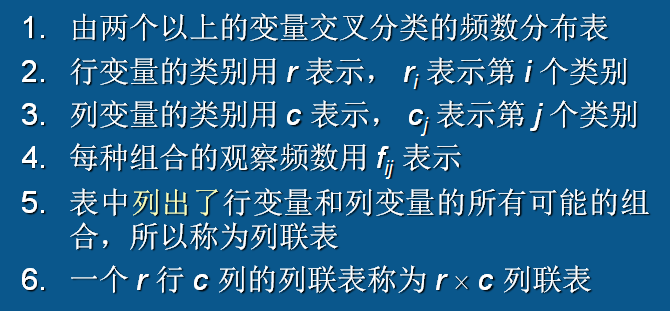二维列联表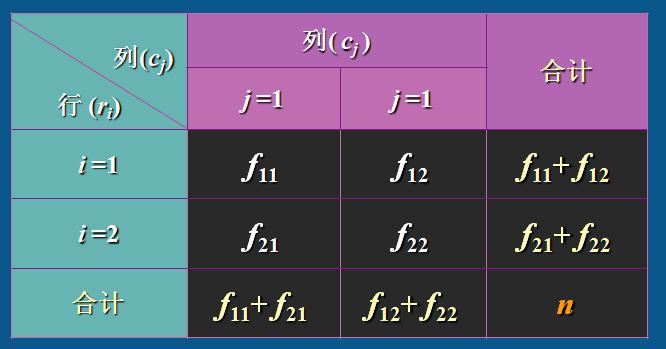r * c 列联表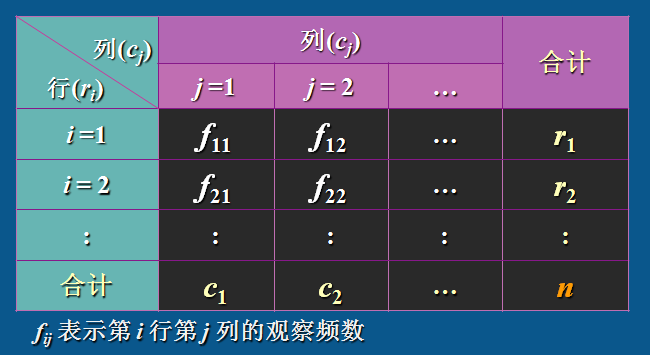观察值的分布百分比分布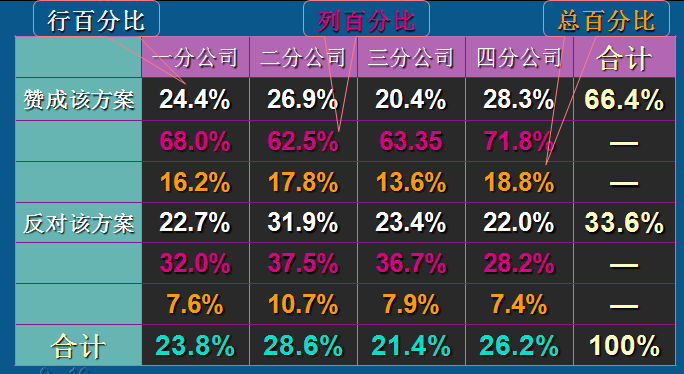期望频数的分布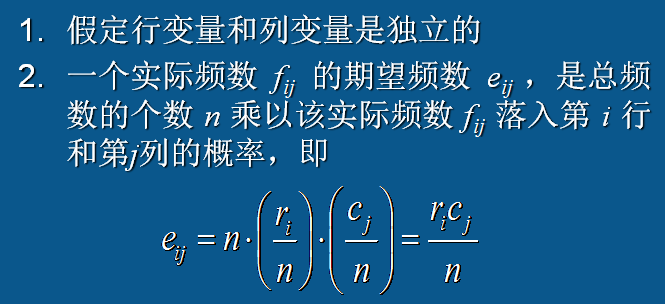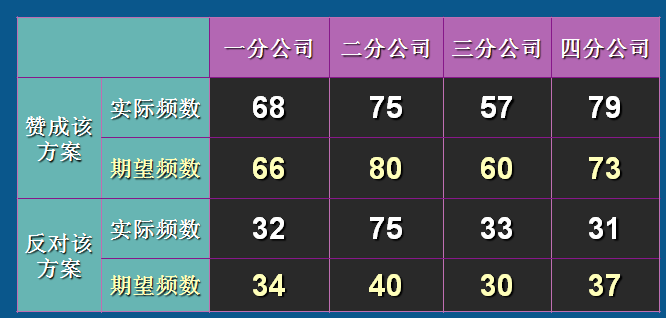假设检验 独立性检验 假设观察频数与期望频数没有差别，而统计量χ2值表示二者间的偏离程度。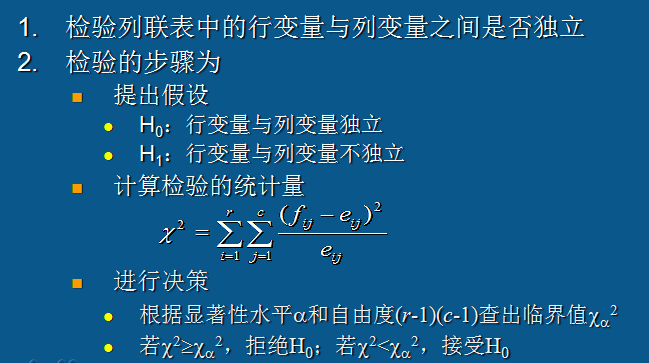相关系数 ψ相关系数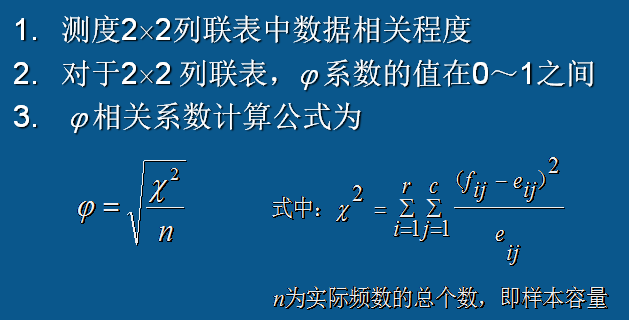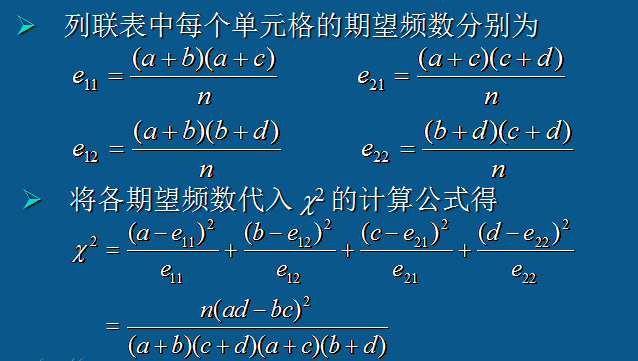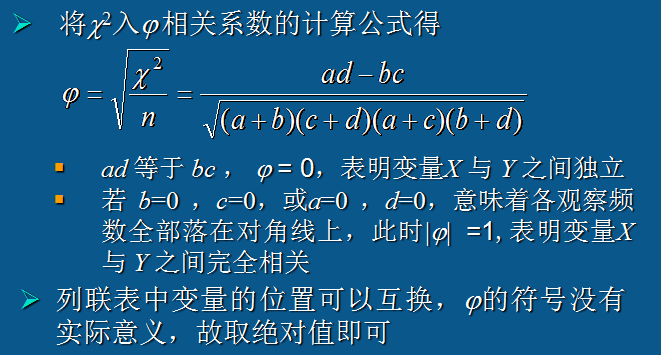皮尔逊定义的列联系数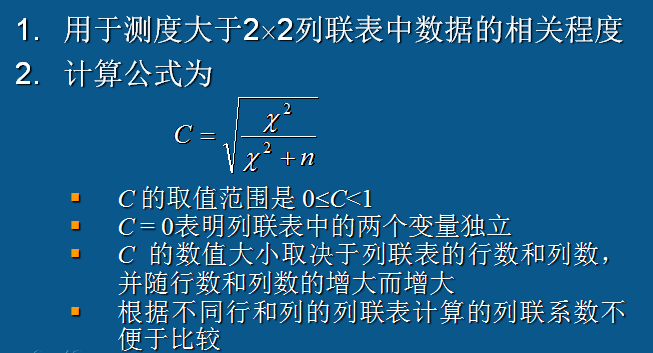V相关系数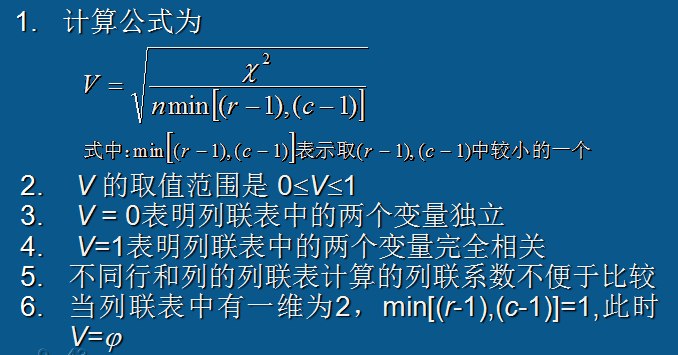Fisher精确检验 卡方统计量是近似的，而Fisher精确检验使用的是超几何分布。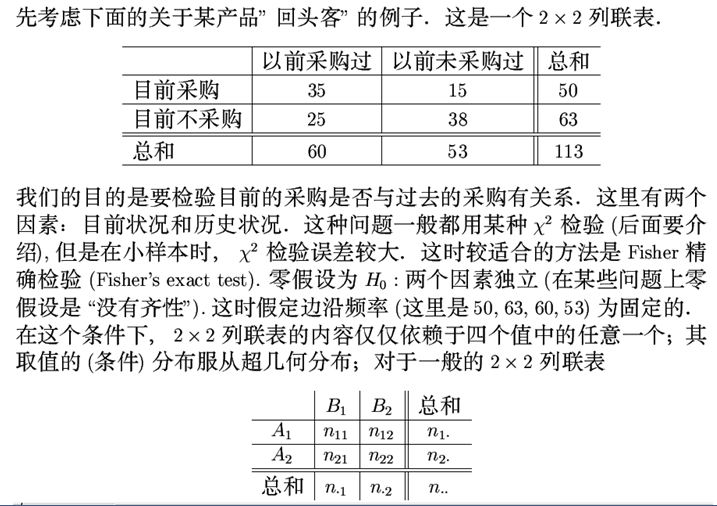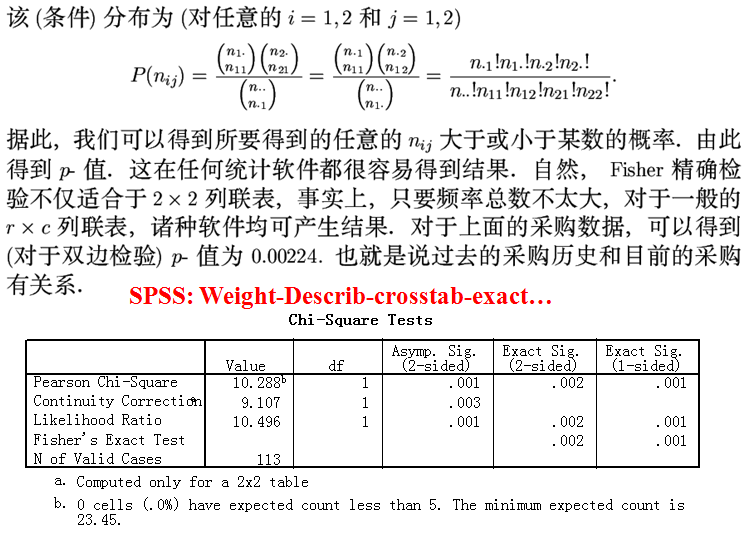相对危险度（Relative Risk, RR） 参考下面的SPSS实例 优势比(Odds Ratio, OR) 参考下面的SPSS实例 Kappa一致性检验 在数据分析中，比较两种预测方法预测结果的一致性用到Kappa检验。 配对χ2检验 通过Kappa检验，解决了两种测量间究竟有无关联的问题，但是通过列联表的观察，发现两位顾问的评价是否不太一致，这种假设又如何来加以分析呢？ McNemar配对χ2检验 就是经典的配对检验，专门用于解决这类问题。 分层χ2检验 分层χ2检验是把研究对象分解成不同层次，按各层对象来进行行变量与列变量的独立性研究。Statistics中Cochran’s and Mantel-Haenszel statistics会自动给出结果。 分层χ2检验是一种很好的控制其他因素的方法，使分析者能得到更准确的结果。如果数据量足够大 ，还可以引入更多的分层因素加以控制。 但是，和SAS中的CMH χ2不同，SPSS提供的CMH χ2检验只能进行二分类变量的检验，而不能进行多分类变量的检验。 检验比较 χ2检验 假设观察频数与期望频数没有差别，而统计量χ2值表示二者间的偏离程度。 卡方检验方法的适用条件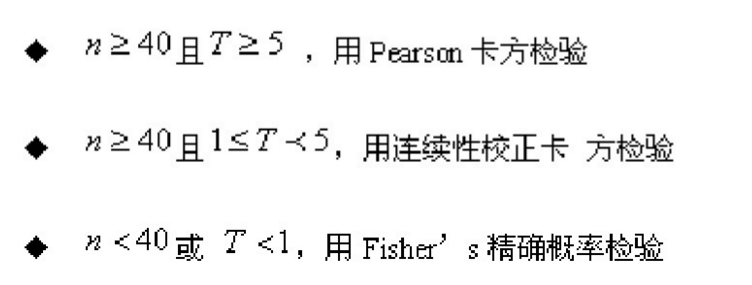关联程度的度量 χ2检验从定性的角度分析是否存在相关行，而各种关联指标（相对危险度RR与优势比OR）从定量的角度分析相关的程度如何。 Kappa一致性检验与配对χ2检验 Kappa一致性检验对两种方法结果的一致程度进行评价，而配对χ2检验则用于分析两种分类方法的分类结果是否有差异。 分层χ2检验 分层χ2检验是把研究对象分解成不同层次，按各层对象来进行行变量与列变量的独立性研究。Statistics中Cochran’s and Mantel-Haenszel statistics会自动给出结果。 SPSS分析 菜单 Analyze -> Descriptive Statistics -> Crosstabs 实例一：卡方检验和风险评估 数据集（site.sav） 某公司实行数据库营销，其杂志销售部每个月向数据库中的人们发送征订邮件，但是回应率极低。他们希望找到一种好的方法来定位潜在的客户，只向这些客户发放邮件，从而节省人力物力。数据库中的资料包括：个人一般信息（年龄、性别、婚姻状况、收入、受教育水平及是否退休等），个人行为特征（主要交通工具、有无手机、呼机、电视、CD及是否订阅报纸）。另外，在发送邮件后，还有一个变量也加入到了数据库中：是否对邮件进行回应，即是否在邮件的提示性进行杂志购买。经研究发现，报纸订阅与邮件发送有相关性。该部门经理想了解报纸订阅者回应邮件的概率是非订阅者的几倍。 参数设置统计量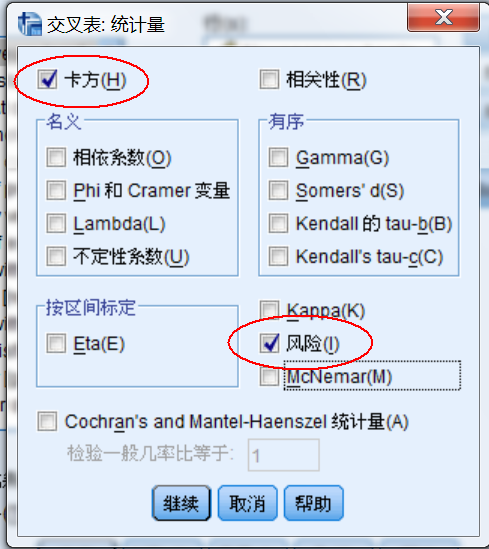结果分析 交叉制表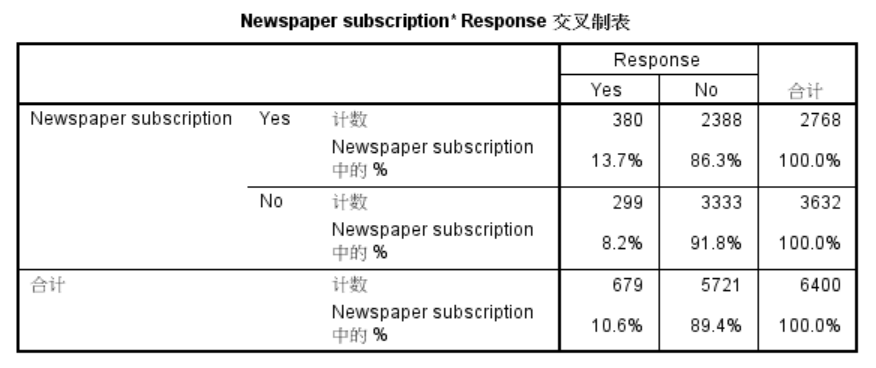列联表分析表明，并没有太多人对杂志的邮件做出回应，但是其中订阅人占了较大比例。 卡方检验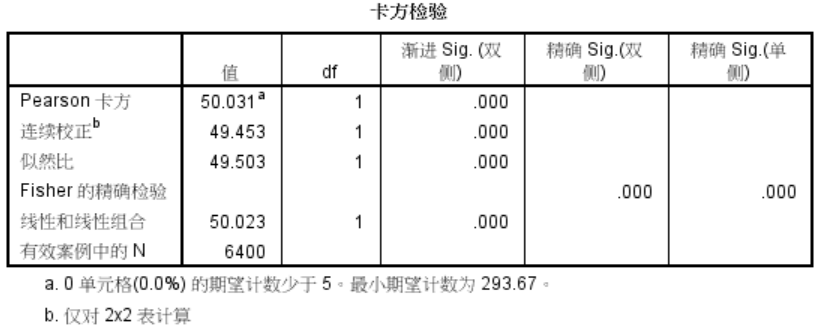p值为0.000，故认为订阅报纸与邮件回应是相关的。那么报纸订阅者的回应概率是未订阅者的多少倍呢？通过计算RR来解决。 风险估计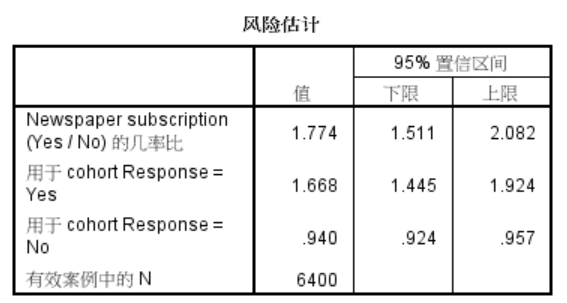对于报纸订阅者而言，邮件响应的相对危险度是其回应概率与非报纸订阅者的回应概率的比值，其估计值是(380/2768) / (299/3632) = 13.7% / 8.2%=1.668，表明报纸订阅者对邮件的响应概率是非报纸订阅者的1.668倍。 或者说报纸订阅者对邮件的无响应的概率是非报纸订阅者的0.94倍。 而优势比即一个事件的Odds Ratio是它发生的概率除以不发生的概率。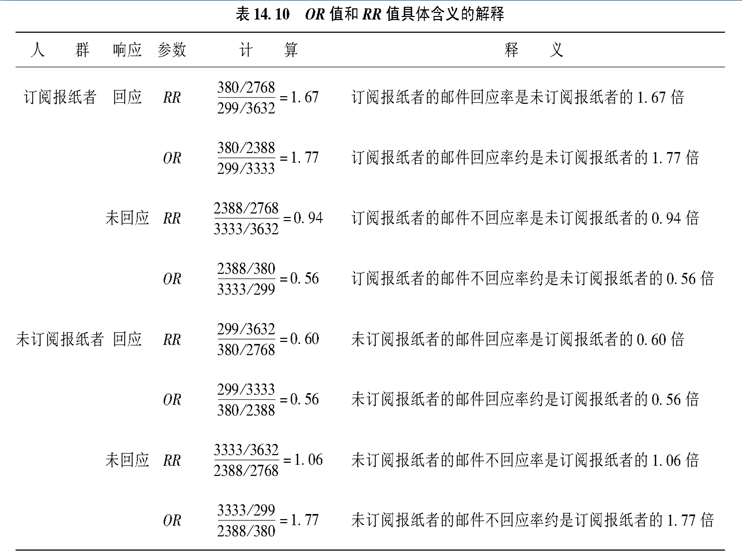实例二：Kappa一致性检验和配对卡方检验 数据集（site.sav） 某公司期望扩展业务，增开几家分店，但对开店地址不太确定。于是选了20个地址，请两位资深顾问分别对20个地址作了一个评价，把它们评为好、中、差三个等级，以便确定应对哪些地址进行更进一步调查，那么这两位资深顾问的评价结果是否一致。 参数设置统计量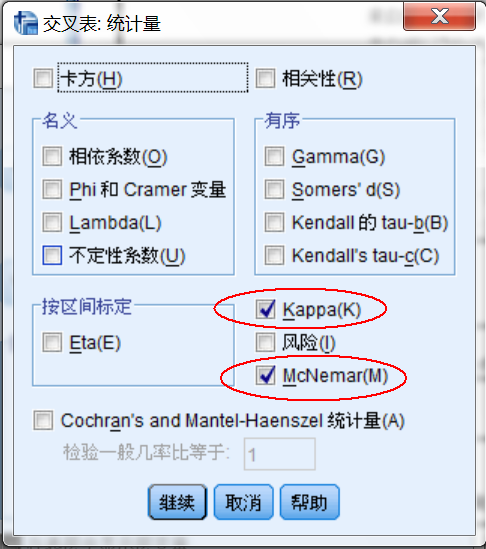结果分析 交叉制表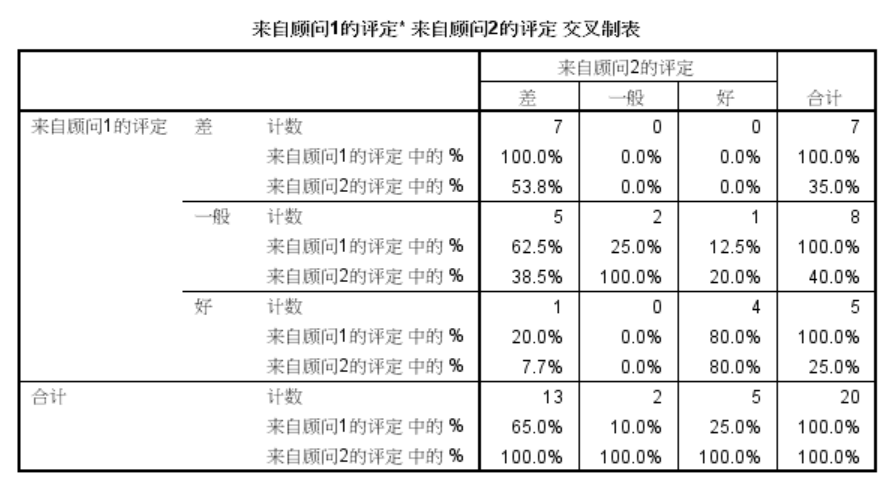Kappa一致性检验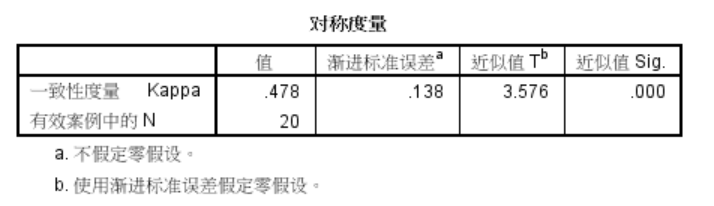Kappa检验的原假设：Kappa=0，即两者完全无关。结果显示Kappa=0.478，P<0.05，拒绝原假设，认为两位顾问的评价结果存在一致性。 配对卡方检验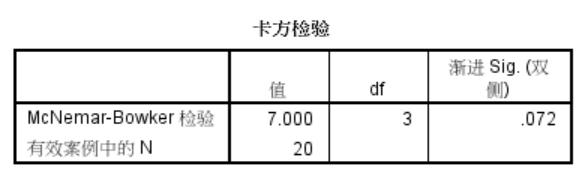Kappa一致性检验对两种方法结果的一致程度进行评价，而配对χ2检验则用于分析两种分类方法的分类结果是否有差异。 此处原假设：两顾问的评价结果无差别，而p=0.072>0.05，故接受原假设，认为基本上相同 实例三：分层卡方检验 数据集（cmh.sav） 某零售连锁店对3家分店的客户满意度进行了调查，数据见cmh.sav，其中一项指标是在购物时是否经常向店员寻求帮助，现希望分析寻求帮助与性别有无联系。 统计结果 未分层的卡方检验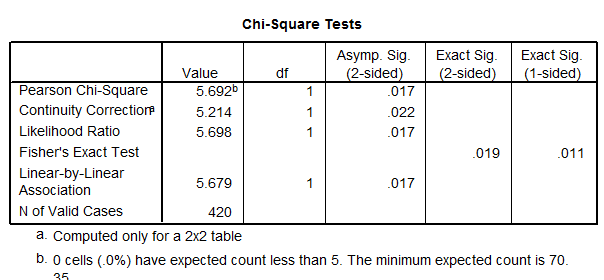将gender和contact分别作为行变量和列变量，并做χ2检验，p<0.05，认为两者间有联系。 因为每家分店的结果可能不一样，上面的卡方检验收到分店因素的影响可能不准确，需要根据分店进行分层统计。 但是分层因素在几个组之间的分布不均，既可能削弱了原本存在的行变量与列变量间的关系，也可能使得原本不存在关系的两个变量关系呈现统计学显著性。 按分店分层卡方检验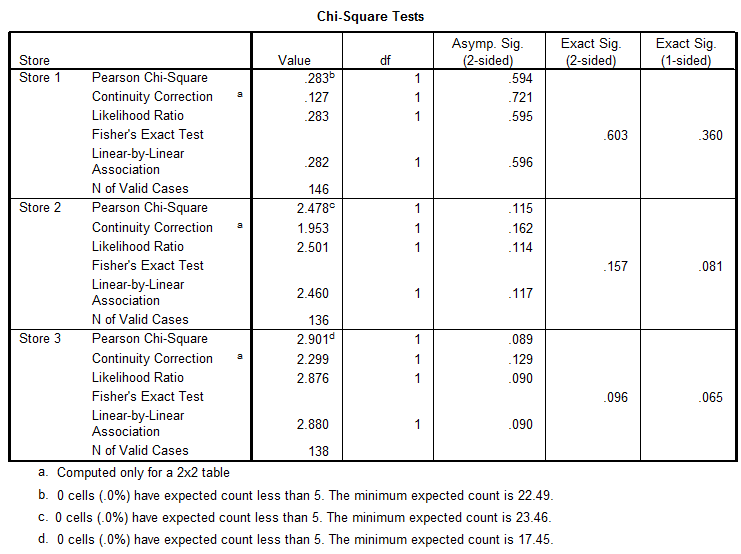可以看到分店的卡方检验并无显著性（p > 0.05），说明每个分店的寻求帮助与性别之间没有强关联。 但是，由于分层后样本量大大减小，这究竟是因为检验效能不足导致的无差异，还是真的无差异？ 为此可以使用Cochran’s and Mantel-Haenszel χ2检验来分析。这种方法可以在考虑了分层因素的影响后给出检验结果。 Cochran’s and Mantel-Haenszel χ2检验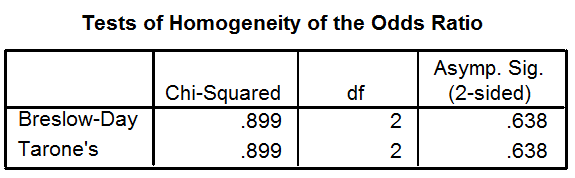首先给出的是层间差异的检验，即考察不同层间gender与contact的联系是否相同。 原假设H0: 分店之间的联系是相同的。 p = 0.638说明，在不同分店层间， gender与contact的联系是相同的。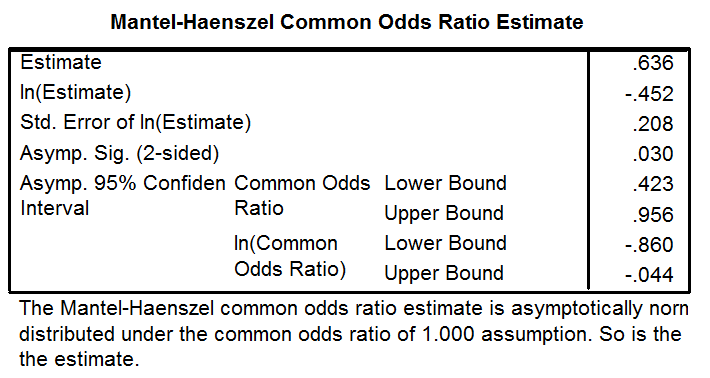调整了分层因素作用后的综合OR值=0.636，即去除了不同分店的混杂效应后，和女性相比，男性顾客寻求帮助的优势比为0.636，或者说更不容易寻求帮助。  展开全文• ## 列联表与卡方分析 千次阅读 2019-09-02 21:24:14 卡方分析用于比较不同组之间的构成比，它的零假设是假定各组之间的构成是相同的，计算出理论每组的理论构成比，再计算理论值与实际值的差别，如果差别大的话...与卡方分析有关的数据形式主要有四格检验，无序RC...R 卡方检验 • 列联表是医学科研中最常见的数据存储格式(或者说数据类型)之一。通常，列联表的横纵方向展示的是两个不同的分类变量，最常见的类型就是四格表(即2×2的列联表)。如下图所示，横向变量是“是否患肺癌”，纵向变量是... • ## 2 X 2 列联表分析 千次阅读 2011-03-10 18:33:00 列联表在统计中运用很广泛，尤其是在分类数据方面，2x2列联表是其中最基础的。...概况起来该种列联表分析法可以描绘的情况有： 1.数据由（分层）随机抽样获得，样本来自两组独立的服从二项分布的总体system • IBM SPSS Statistics的列联表分析，也称为交叉表分析，用于分析两个或以上分组变量的相关关系，在分析影响满意度的因素、药物有效性等方面都有很好的应用。 本文将以较为简单的二乘二列联表卡方检验为例，介绍一下... • 为了研究性别与满意度的相关关系，如图1所示，我们需要分别将性别、满意度变量添加到右侧的行、列中，形成二乘二的列联表。 图1：选择变量 二、设置精确检验方法 然后，再单击“精确”选项，设置分析所用的... • *一，随机设计四格; options validvarname=any; data test1; input 用药$ 敏感性 \$ 计数; datalines; 服药 不敏感 180 服药 敏感 215 未服药 不敏感 73 未服药 敏感 106 ; proc freq data=test1 order=data; ...
• 以顾客这个对象为例子，它可以按性别分类为男女，也可以按单次消费金额分类为高，中，底几个档次，通过列联表相关分析方法将能够获知顾客的性别与单次消费金额档次是否存在关联，它得到的是简单的结论，例如顾客...
• 列联表也是频数表，只不过它会分析的是将数据集按两个或两个以上类别变量联合分组时观察数据在每个分组中出现频数的表，所以又称交叉分类表。 简介 特殊地，如果我们使用样本数据的两个属性构建列联表，而且每个...
• ## 列联分析

千次阅读 2020-04-06 11:21:18
列联分析是利用列联表来研究() A、两个分类变量的关系 B、两个数值变量的关系 C、一个分类变量一个数值变量 C.两个数值型变量的分布 正确答案： A 2 设R为列联表的行数,C为列联表的列数,则χ2分布自由度为() A、R B...统计学
• 列联表也是频数表，只不过它会分析的是将数据集按两个或两个以上类别变量联合分组时观察数据在每个分组中出现频数的表，所以又称交叉分类表。简介特殊地，如果我们使用样本数据的两个属性构建列联表，而且每个属性...
• 1.列联表是按两个或多个特征分类的频数数据，一般以表格形式组成。 2.判断两个或多个属性之间有无关联，即判别属性之间是否独立。 3.检验步骤 建立原假设 H0: 两属性相互独立 H1: 两属性之间不独立 计算自由度 ...
• 一、相关分析相关分析是什么？有哪些分类？各类相关分析的用途是什么？ 相关分析是研究现象之间是否存在某种依存关系，并对具体有依存关系的现象探讨其相关方向以及相关程度，是研究随机变量之间的相关关系的一种...
• 描述2*2列联表数据相关程度最常用的一种相关系数，  n为列联表中的总频数，也即样本量 几种情况说明 a、当ad=bc， 为0，表示X,Y之间互相独立 b、b=...R 独立性检验
• H1：两变量相互独立 Step2：计算自由度与理论频数 Step3：计算统计量 Step4：查χ2方分布临界值，确定接受域例：对表1所示频数分布，以95%显著水平，检验色觉与性别是否有关。解： Step1：H0：色觉与性别...
• 2019独角兽企业重金招聘Python工程师标准>>> ...c、如果列联表中有一维为2则min[（R-1）,（C-1）]=1，则V=   转载于:https://my.oschina.net/u/1785519/blog/1430993
• 1、当列联表R*C中的行数R或列数C大于2时 2、此时 系数会随着R或C增大而增大，且没有上限，所以用 系数测定两个系统的相关程度就不够清晰 可采用列联相关系数 列联相关系数 ...
• 分析数据时，需要通过一些工具，把数据转换成，我们能直观理解处理的数据，频数表和列联表比较容易理解； 生成频数表方法 函数 描述 table 使用N个类别型变量（因子）创建一个N维列联表，向量是列变量 ...R
• 前天在看书的时候第一次看到了列联表卡方检验，觉得这个东西不难又相对容易实现，刚好知乎上有个老哥的文章是用R来实现卡方检验，于是借用他的数据，我在spyder上面实现了。 这是一份手游数据，里面是某手游2013年8...编程语言 Python
• ## 第九章 列联分析

千次阅读 2015-08-31 19:42:09
列联分析主要用于分类数据的分析1 分类数据与列联表1 分类数据如：完整家庭/离异家庭、一等品/二等品、三等品……2 列联表的构造列联表是由两个以上的变量进行交叉分类的频数分布表。3 列联表的分布列联表的分布可以...
• 由现有汇总表生成列联表的例子： 例2.3 下表记录了某公司在过去6个月中的顾客信息.其中包括顾客的性别(GEN:0=男和1=女)，顾客的年龄(AGE),顾客的年收入(INCLEV：1=低,2=中和3=高)和购买价值(PURCHASE：0=小于100元,...
• 相关系数图 library(vegan) library(dplyr) library(corrplot) par(omi = c(0.3, 0.3, 0.3, 0.3), cex = 1.2, family = ‘Times New Roman’) # windows系统可能需要安装其他字体包 M <- cor(decostand(mtcars,...可视化
• Others列联表多重插补 Python手册(Machine Learning)–statsmodels(Quickstart) Python手册(Machine Learning)–statsmodels(Regression) Python手册(Machine Learning)–statsmodels(TimeSeries) Python手册...
• 本节书摘来自华章计算机《数学建模：基于R》一书中的第1...1.5　列联表检验 设两个随机变量X,Y均为离散型的,X取值于{a1,a2,…,aI},Y取值于{b1,b2,…,bJ}.设(X1,Y1),(X2,Y2),…,(Xn,Yn)为简单样本,记nij为(X1,Y1),(X......